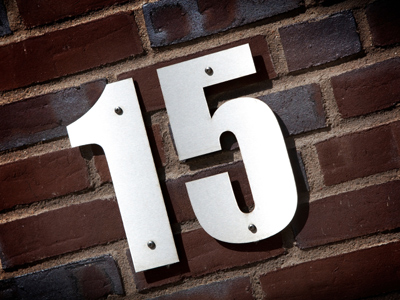5 x 3 = 15.

# 3 Times Table

This Math quiz is called '3 Times Table' and it has been written by teachers to help you if you are studying the subject at elementary school. Playing educational quizzes is an enjoyable way to learn if you are in the 3rd, 4th or 5th grade - aged 8 to 11.

It costs only \$12.50 per month to play this quiz and over 3,500 others that help you with your school work. You can subscribe on the page at Join Us

Learning the 3 times table involves multiplying numbers by 3. Knowing your times tables helps with all kinds of math. Even if you get all the questions right first time keep on taking the quiz as you can improve on the time it takes you to answer all the questions. Learning your times tables will make multiplying numbers, and so your math lessons, a lot easier!

Test your knowledge of the 3 times table by playing this quiz.

1.
7 x 3 =
21
23
25
30
2.
2 x 3 =
3
5
6
9
To times a number by 2 just double it
3.
8 x 3 =
11
20
24
26
There are 24 hours in a day
4.
4 x 3 =
10
12
14
15
5.
12 x 3 =
12
30
36
15
6.
9 x 3 =
12
19
26
27
To multiply a number by 9 just times it by 10 then take it away, so 3 x 10 = 30, 30 - 3 = 27
7.
5 x 3 =
10
15
18
22
15 is 3 + 3 + 3 + 3 + 3 and 5 + 5 + 5
8.
11 x 3 =
15
33
14
35
9.
6 x 3 =
9
18
22
24
10.
3 x 3 =
3
6
8
9
A number multiplied by itself is known as a square number, so 9 is 3 squared
Author:  Amanda Swift